# Texas Go Math Kindergarten Lesson 10.7 Answer Key Decompose 10

Refer to our Texas Go Math Kindergarten Answer Key Pdf to score good marks in the exams. Test yourself by practicing the problems from Texas Go Math Kindergarten Lesson 10.7 Answer Key Decompose 10.

## Texas Go Math Kindergarten Lesson 10.7 Answer Key Decompose 10

Essential Question
How do you take away numbers from 10?

Explore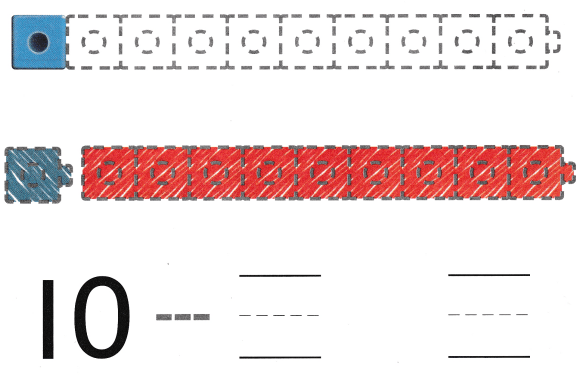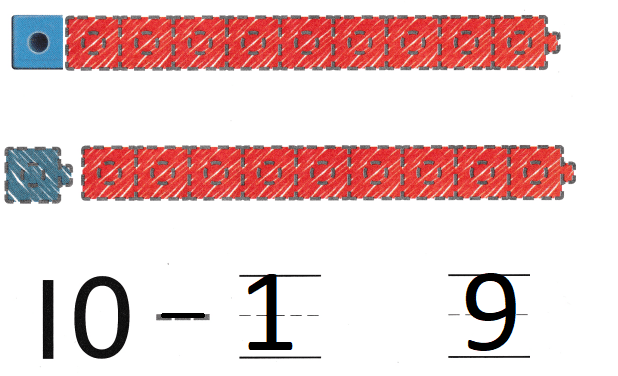Explanation:
a ten-cube train.
One cube is blue and the rest are red.
10 – 1 = 9
so, 9 red cubes.

Directions
Model a ten-cube train. One cube is blue and the rest are red. Take apart the train to model the set taken apart. Draw and color the cube trains. Trace the symbol and write the numbers to show 10 taken apart.

Share and Show

Question 1.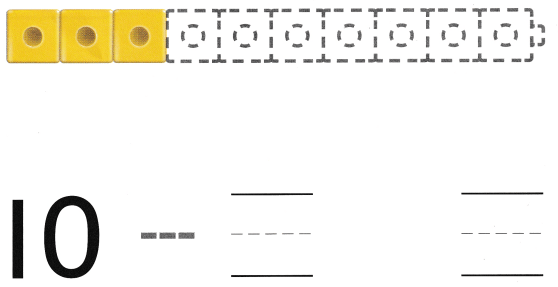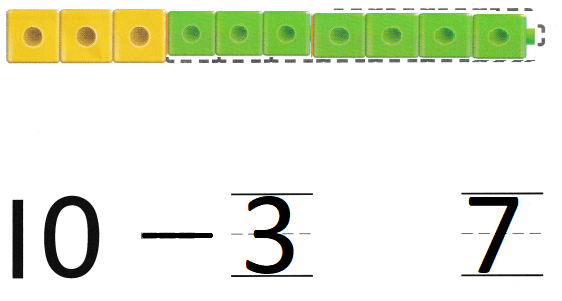Explanation:
a ten-cube train. Three cubes are yellow and the rest are green.
10 – 3 = 7
so, 7 are green cubes.

Question 2.Explanation:
Six cubes are orange and the rest are blue.
10 – 6 = 4
so, 4 are blue.

Directions
1. Model a ten-cube train. Three cubes are yellow and the rest are green. Take apart the train to model the set taken apart. Draw and color the cube trains. Trace the symbol and write the numbers to show 10 taken apart. 2. Model a ten-cube train. Six cubes are orange and the rest are blue. Take apart the train to model the set taken apart. Draw and color the cube trains. Trace the symbol and write the numbers to show 10 taken apart.

Question 3.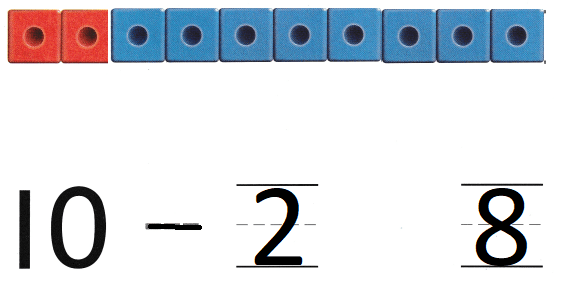Explanation:
a ten-cube train. Two cubes are red and the rest are blue.
10 – 2 = 8
so, 8 cubes are blue

Question 4.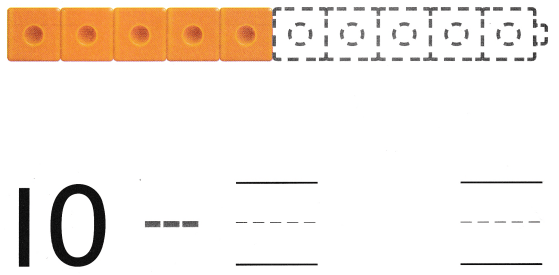Explanation:
In a given ten cube train
Five cubes are orange and the rest are green
10 – 5 = 5
so, 5 are green

Directions
3. Model a ten-cube train. Two cubes are red and the rest are blue. Take apart the train to model the set taken apart. Draw and color the cube trains. Trace the symbol and write the numbers to show 10 taken apart. 4. Model a ten-cube train. Five cubes are orange and the rest are green. Take apart the train to model the set taken apart. Draw and color the cube trains. Trace the symbol and write the numbers to show 10 taken apart.

Home Activity

• Ask your child to show a set of 10 objects, using objects of the some kind that are different in one way, for example, large and small paper clips. Then have him or her write the numbers that show how many of each kind are in the set.

Problem Solving

Question 5.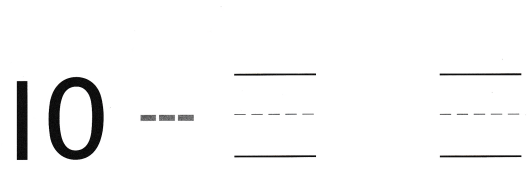Explanation:
There are 10 paper clips
In ten five are large and rest are small
so, 10 – 5 = 5
so, 5 are small paper clips.

Question 6.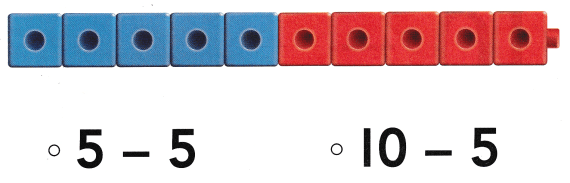Explanation:
10 cube train is given
5 are blue
10 – 5 = 5
so, 5 are red cubes.

Directions
5. Sydney has 10 square tiles. 8 of the tiles are red. The rest are yellow. How many tiles are yellow? Draw the tiles. Write the numbers and trace the symbol. 6. Choose the correct answer. Which does the cube train show?

### Texas Go Math Kindergarten Lesson 10.7 Homework and Practice

Question 1.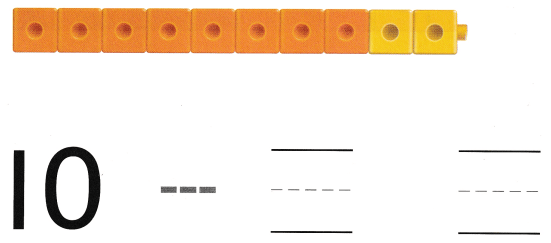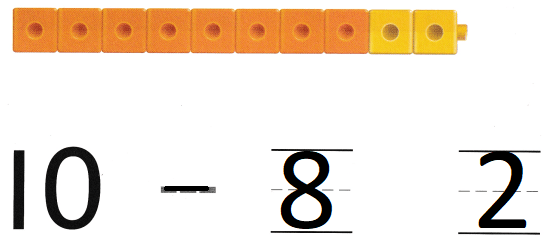Explanation:
the given  ten-cube train.
Eight cubes are orange and the rest are yellow.
10 – 8 = 2
so, 2 are yellow cubes

Question 2.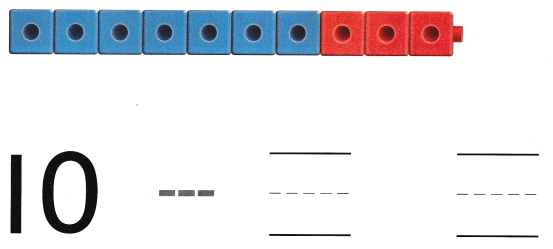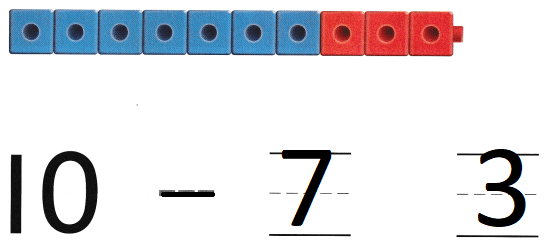Explanation:
10 cube train is given
7 are blue
10 – 7 = 3
so, 3 are red cubes.

Directions
1. Look at the ten-cube train. Eight cubes are orange and the rest are yellow. Take apart the train by drawing to model the set taken apart. Color the cube trains. Trace and write to show 10 taken apart. 2. Look at the ten-cube train. Seven cubes are blue and the rest are red. Take apart the train by drawing to model the set taken apart. Trace and write to show 10 taken apart.

Texas Test Prep

Lesson Check

Question 3.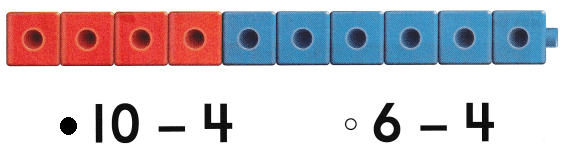Explanation:
Given train is 10  cube train
4 are red
10 – 4 = 6
so, 6 are blue cubes.

Question 4.